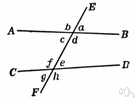# interior angle

(redirected from Interior angles)
Also found in: Thesaurus, Encyclopedia.
Related to Interior angles: exterior angles
interior angle
Angles 3, 4, 5, and 6 are interior angles. Angles 3 and 6 are alternate interior angles, as are angles 4 and 5.

## interior angle

n.
1. Any of the four angles formed between two straight lines intersected by a third straight line.
2. An angle formed inside a polygon by two adjacent sides.

## interior angle

n
1. (Mathematics) an angle of a polygon contained between two adjacent sides
2. (Mathematics) any of the four angles made by a transversal that lie inside the region between the two intersected lines
Collins English Dictionary – Complete and Unabridged, 12th Edition 2014 © HarperCollins Publishers 1991, 1994, 1998, 2000, 2003, 2006, 2007, 2009, 2011, 2014

## inte′rior an′gle

n.
1. an angle formed between parallel lines by a third line that intersects them.
2. an angle formed within a polygon by two adjacent sides.

## in·te·ri·or angle

(ĭn-tîr′ē-ər)
1. Any of the four angles formed inside two straight lines when these lines are intersected by a third straight line.
2. An angle formed by two adjacent sides of a polygon and included within the polygon. Compare exterior angle.
ThesaurusAntonymsRelated WordsSynonymsLegend:
 Noun 1interior angle - the angle inside two adjacent sides of a polygoninternal angleangle - the space between two lines or planes that intersect; the inclination of one line to another; measured in degrees or radiansreentering angle, reentrant angle - an interior angle of a polygon that is greater than 180 degrees
Based on WordNet 3.0, Farlex clipart collection. © 2003-2012 Princeton University, Farlex Inc.
References in periodicals archive ?
What do the interior angles of a square add up to in degrees?
Lateral Cephalometric Measurements Cephalometric Measurement Mean Initial VERTICAL ANALYSIS SN-GoGn 32[degrees][+ or -] 38.1[degrees] 8[degrees] Saddle angle 123[degrees][+ or -] 137.6[degrees] 5[degrees] Articular angle 143[degrees][+ or -] 144.2[degrees] 6[degrees] Gonial angle 130[degrees][+ or -] 112.5[degrees] 7[degrees] Sum of interior angles 396[degrees][+ or -] 394.3[degrees] 3[degrees] Jarabak (SGo-NMe) 59%-63% 63.5% ANS-Me/N-Me 55% 58.4% Max.
We had the lines are parallel then we know the alternate interior angles are congruent.
###demonstrate corresponding angles, alternate interior angles,
* The interior angles follow the pattern (n--2)(180[degrees])/n, where n is the number of sides.
Since m[angle]DPQ = m[angle]PQR, (because trapezium PQC'D' is a reflection of trapezium PQCD along the line segment PQ) and m[angle]DPQ = m[angle]RPQ (because alternate interior angles are congruent when a transversals intersects two parallel lines), APQR is an isosceles triangle with m[angle]PQR = m[angle]RPQ.
Perhaps, if we could have such an acquaintance, we could know directly and unhypothetically that its interior angles sum to two right angles.